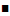The fiscal stimulus packages shifts the AD curve up.    See the AS/AD chapter.Recall the IS curve equation,and the simple monetary policy rulethat are combined to form the AD equation.And what is a ‘fiscal stimulus package’ in this AD equation? It would be a positive shock towhich is in, shifting the AD equation up.